# Calculators for Windows

A long list of basic or scientific calculators that improve the performance and functions of the native Windows calculator when it comes to maths operations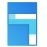Calculator 2020.921.0.0

Carry out the fastest maths calculations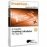DreamCalc 5.0.4 Professional Edition

A complete calculator for any task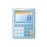A complete and attractive calculator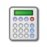Mathematical calculations within everyone's grasp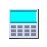HEXelon MAX 6.07.08

Finish complicated math calculations very quickly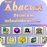Calculus program developed for chemists, physicists and architects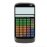MyCalc2 1.20

Totally fantastic free calculator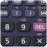Khi3 3.4.8

Complete scientific calculator with plenty of options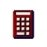Useful calculator similar to that of Windows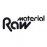Practical weight, area and price calculator for materials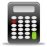Calq 1.3.1

A basic calculator that is always available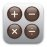OpalCalc 1.90

Multiline calculator that integrates natural language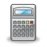ESBCalc 7.3.1

Very complete calculator with scientific options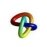Solve matrices in the quickest and simplest way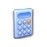A much more complete version of the Windows Calculator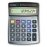Popular calculator with a currency converter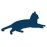Easily convert measurement unitsConverber 2.3.1

Convert between different units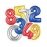OfiCalc 10.1

Incredible scientific calculator that also include a personal organizer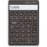A very simple formula calculator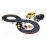Measure the length of your slot tracks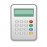Calculator conceived to calculate time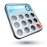ATCalc 4.0

Customizable scientific and graphic calculator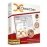Deskcalc 8.3.8

One of the best alternatives to Windows calculator